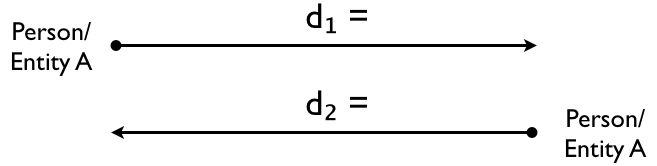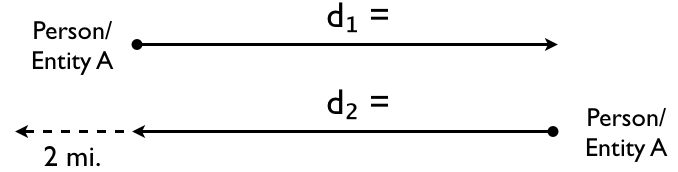Learn the 4 Things You Must Know Before Taking the GMAT!   Download it Now!

# Motion Problems: Round-Trip

Motion problems are among the most common word problems you’ll encounter on the Quantitative section of the GMAT. These problem solving questions fall into one of three categories:

1. Motion in Opposite Directions
2. Motion in the Same Direction (“Catch Up” Problems)
3. Round Trip Questions

In this lesson we’ll cover Round-Trip Motion Questions.

### 3. Round-Trip Motion: The Basics

All types of motion questions on the GMAT require you to apply the following basic Distance Formula:

Distance = Rate * Time

The general idea with Round Trip motion questions is that you have an entity (car, person, train, etc.) heading out in one direction at a certain rate of speed, and then returning along the same route at a different rate of speed, often after delaying for a certain amount of time before starting the return journey. The question itself may ask you any number of things, including what the average rate of speed is for the entire trip or how much distance the journey took given a certain total elapsed time.

Regardless of what information is given to you and what you’re ultimately asked to solve for, these questions always come down to solving for one of the variables in the Distance Formula. There are three crucial steps to finding the missing piece that the question is asking you to solve for.

### The Strategy

Step 1: Draw a diagram illustrating what the problem is telling you. This will help you visualize what you’re solving for and get your mind around the question itself.

The diagram will usually look something like this:Step 2: The best starting point for solving Round Trip questions is to use this formula:

d = d₂

Step 3: Set up an individual distance formula for each leg of the trip, d₁ and d₂, and set them equal to each other.* You may be asked to find the distance traveled in a certain amount of time, or perhaps you’ll simply be asked what the average rate is for the trip.

* Note: On harder questions, the Person/Entity may return and go past where he/she started, or some other similar variation. In that case, obviously d₁ and d₂ will not be equal. You’ll want to use your diagram as a guide and adjust your equation as necessary. For example, if the problem asks you what the average rate of speed for the trip is if the Person/Entity returns along the same route and goes past his starting point to a friend’s house that is 2 miles further down the road, then your diagram may look like this:Thus, for those two distances to be “equal,” you’ll have to adjust your equation as follows (assuming the rate is given in miles):

d + 2 = d₂

In other words, the Person/Entity would in theory have had to have started the journey 2 miles further down the road (or gone 2 miles further on the trip out) in order for the distance “out” to equal the distance “back,” hence the reason we add 2 to d₁.

### On the GMAT

This will all make more sense once you see how this strategy is applied to a sample GMAT question. Watch this video for a step-by-step application: